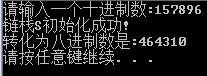# 数据结构栈数制转换

## 数据结构-栈的实现之数制转换

2016-04-12 21:08:00 weixin_30905981 阅读数 13

``` 1void conversion(int a, int b)　　//参数为从a进制转换为b进制 2 {
3     int num = 0;
4     char z = 'A';
5     printf("请输入你想要转换的%d进制数",a);
6     scanf("%d", &num);
7     while(num)
8     {
9         Push(Scale, num%b);　　//取余数逐个进栈
10         num /=b;　　//求商
11     }
12     int e = 0;
13     printf("转换为%d进制数为：", b);
14     while(!StackEmpty(Scale))
15     {
16         Pop(Scale, e);　　　//逐个出栈
17         if(e >= 10 && e <= 15)　　//结果为十六进制时用到转换为字母
18             printf("%c", z+e-10);
19         else
20             printf("%d", e);
21     }
22     printf("\n");
23 }```

## 数据结构 利用栈进行数制转换的实现

2019-04-13 20:05:22 qq_44713855 阅读数 160

#include
#include
#define STACK_INIT_SIZE 100
#define STACKINCREMENT 10
typedef int ElemType;
typedef int Status;
using namespace std;
typedef struct
{
int *base;
int *top;
int stacksize;
}SqStack;
Status initStack(SqStack &S)
{
S.base = (ElemType *)malloc(STACK_INIT_SIZE * sizeof(ElemType));
if (!S.base)
{
cout << “内存分配失败!” << endl;
exit(0);
}
S.top = S.base;
S.stacksize = STACK_INIT_SIZE;
return 1;
//判断栈是否为空
}
Status push(SqStack &S, ElemType e)
{
if (S.top - S.base >= S.stacksize)
{
S.base = (ElemType *)realloc(S.base, (S.stacksize + STACKINCREMENT) * sizeof(ElemType));
if (!S.base)
{
exit(0);
}
S.top = S.base + S.stacksize;
S.stacksize += STACKINCREMENT;
}
*S.top++ = e;
return 1;
}//插入元素e为栈顶元素
Status StackEmpty(SqStack S)
{
if (S.top == S.base)
return 1;
else
return 0;
}

Status pop(SqStack S, ElemType &e)
{
if (S.base == S.top)
{
cout << “栈为空！” << endl;
return 0;
}
e = *–S.top;
return 1;
}//删除栈顶元素，并返回
int main()
{
ElemType e;
SqStack S;
initStack(S);
int N;
cin >> N;
while (N)
{
push(S, N % 8);
N /= 8;
}
while (!StackEmpty(S))
{
pop(S, e);
cout << e;
}
cout << endl;
system(“pause”);
return 1;
}

## 数据结构—栈的运用之数制转换

2019-07-04 20:37:08 qq_22847457 阅读数 452

1.案例分析

• 当将一个十进制整数N转换为八进制数时，在计算过程中，把N与8求余得到的八进制数的各位依次进栈，计算完毕后将栈中的八进制数依次出栈输出，输出结果就是待求得的八进制数。

2.算法步骤

• ①初始化一个空栈S。
• ②当十进制数N非零时，循环执行以下操作:
• 把N与8求余得到的八进制数压入栈S;
• N更新为N与8的商。
• ③当栈S非空时，循环执行以下操作:
• 弹出栈顶元素e;
• 输出e

3.算法描述

``````void conversion(int N)
{//对于任意一个非负十进制数，打印输出与其等值的八进制数
int e;
if (InitStack(S))
{
printf("链栈S初始化成功!\n");
}
else
{
printf("链栈S初始化失败!\n");
}
while (N) //当N非零时,循环
{
Push(S, N % 8);    // 把N与8求余得到的八进制数压入栈S
N = N / 8;      // N更新为N与8的商
}
printf("转化为八进制数是:");
while (S) // 当栈S非空时，循环
{
Pop(S, e); //弹出栈顶元素e
printf("%d", e); //输出e
}

}``````

4.代码实现

• main.cpp
``````#include <iostream>

using namespace std;

// 链栈的存储结构
typedef struct StackNode
{
int data;
struct StackNode *next;

// 初始化
{
S = NULL;   // 将栈顶指针置空
return 1;
}

// 入栈
{
//元素e入栈
StackNode *p;
p = new StackNode;  // 生成新节点

p->data = e;        // 将新节点数据域置为e
p->next = S;        // 将新节点插入栈顶
S = p;             // 修改栈顶指针
return 1;         // 链栈要注意指针的方向是从栈顶指向栈底的
}

// 出栈
{
if (S == NULL)
{
return 0;    // 栈空
}
e = S->data;    //将栈顶元素赋值给e
StackNode *p;
p = S;         // 临时保存栈顶元素空间，准备释放
S = S->next;    // 修改栈顶指针
delete p;     // 释放原栈顶元素空间
return 1;
}

// 数制的转换(链栈实现)
void conversion(int N)
{//对于任意一个非负十进制数，打印输出与其等值的八进制数
int e;
if (InitStack(S))
{
printf("链栈S初始化成功!\n");
}
else
{
printf("链栈S初始化失败!\n");
}
while (N) //当N非零时,循环
{
Push(S, N % 8);    // 把N与8求余得到的八进制数压入栈S
N = N / 8;      // N更新为N与8的商
}
printf("转化为八进制数是:");
while (S) // 当栈S非空时，循环
{
Pop(S, e); //弹出栈顶元素e
printf("%d", e); //输出e
}

}

int main()
{
int n;

printf("请输入一个十进制数:");
scanf("%d", &n);

conversion(n);
printf("\n");

system("pause");

return 0;
}``````
• 运行结果## 数据结构-栈-数制转换

2017-03-28 13:39:09 zhongcanw 阅读数 269

`	void  exchange(int value,int n)`
`	{`
`		stack<int> s;`
`		while(value>0)`
`		{`
`			s.push(value%n);`
`			value=value/n;`
`		}`
`		while(!s.empty())`
`		{`
`			`
`			cout<<s.top();`
`			s.pop();`
`		}`
`	}`

## 数据结构—栈的应- 数制的转换

2018-07-25 10:56:14 wu_comet 阅读数 216

//因为数值转换的最后的结果是逆序排列，故可以用堆来实现

//这里用静态的顺序栈来实现数制的转换

include<iostream>
#include<stdio.h>
using namespace std;

#define max_stacksize 200

typedef int ElemType;
typedef struct SqStack{
ElemType Stack_arry[max_stacksize];
int top;
}SqStack;

void init_stack(SqStack &s){         //初始化协议栈
s.top=-1;
}

int push(SqStack &s,int x){         //进栈操作
if(s.top>=max_stacksize-1){
printf("栈满！");
}
else{
s.Stack_arry[++s.top]=x;
}
return 0;
}

int pop(SqStack &s,int &x){        //退栈操作
if(s.top==-1){
printf("栈空！");
}
else{
x=s.Stack_arry[s.top--];
}
return x;
}

void conversion(int n,int d){  //将n进制的数转化为d进制的数
SqStack s;
init_stack(s);
int b,e;
while(n!=0){
b=n%d;             //求余
n=n/d;               //求商
push(s,b);

}
while(s.top!=-1){
pop(s,e);
printf("%d",e);
}
}

int main(){
int N,D;
cin>>N>>D;
conversion(N,D);
return 0;
}